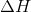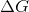# AP Chemistry : Reactions and Equilibrium

## Example Questions

← Previous 1 3 4 5 6 7 8 9 18 19

### Example Question #1 : Reaction Types

What is the balanced equation when heptane is combusted?

2 C7H14 + 5 O2 → 3 H2O + 6 CO2

C7H16 + 11 O2 → 8 H2O + 7 CO2

C5H12 + 8 O2 → 6 H2O + 5 CO2

C7H16 + O2 → H2O + CO2

C3H8 + 5 O2 → 4 H2O + 3 CO2

C7H16 + 11 O2 → 8 H2O + 7 CO2

Explanation:

Heptane: C7H16. Combustion is when a molecule reacts with O2 and the products are CO2 and H2O. Balancing gives 7 CO2, 8 H2O, 1 heptane, and 11 O2

### Example Question #2 : Reaction Types

Which of the following reactions has the most exothermic heat of reaction?

The combustion of isobutane
The combustion of methane
The combustion of butane
The combustion of propane
The combustion of isopropane
Correct answer: The combustion of butane
Explanation:

The longer the hydrocarbon chain, the greater the amount of combustion products (CO2 and H20) generated. Branched molecules such as isopropane and isobutane are more difficult to combust than their straight-chain counterparts.

### Example Question #3 : Reaction Types

Which of the following conditions would describe a combustion reaction?

Exothermic with a positiveEndothermic with a positiveExothermic with a negativeExergonic with a positiveEndergonic with a negativeExothermic with a negativeExplanation:

An exothermic reaction will have a negativevalue, indicating that it releases heat. Conversely, an endothermic reaction will have a positivevalue, indicating a consumption of heat.

A combustion reaction releases heat; thus it must have a negativevalue and be exothermic.

Exergonic reactions have a negativevalue, indicating spontaneity, while endergonic reactions are non-spontaneous. While most combustion reactions will be non-spontaneous, it is impossible to draw this conclusion for certain without knowing more about the reaction. The only thing we know for certain is that heat is released, and the reaction is exothermic.

### Example Question #1 : P H

What is the pH of a solution that has [OH-] 1 X 10–4 M?

8

6

-4

4

10

10

Explanation:

pOH would be 4 (use –log [OH–]) and pH would be 14–pOH = 14 – 4 = 10

### Example Question #5 : Reaction Types

What is the pH of a solution with [OH-] = 4 X 10-6

12.1

2.2

8.6

4

6

8.6

Explanation:

[OH-] = 4 X 10-6

pOH = 5.4 — use –log [OH] to find pOH

pH = 14– pOH = 8.6

### Example Question #1 : P H

What is the pH of a soution containing .0001 M HCl?

3

6

5

7

4

4

Explanation:

The pH of a solution is determined by taking the negative log of the concentration of hydrogen ions in solution.  HCl is strong acid so it completely dissociates in solution.  So adding .0001 M HCl is the same as saying that 1 *10-4 moles of H+ ions have been added to solution.  The -log[.0001] =4, so the pH of the solution =4.

### Example Question #7 : Reaction Types

Which of the following solutions contains the greatest number of H+ ions?

5 mL of NaOH

1.0 mL of NaCl in excess water

0.010 mL of KOH in excess water

0.010 mL of HCl in excess water

0.010 mL of CH3OH in excess water

0.010 mL of HCl in excess water

Explanation:

This question asks for the solution with the greatest number Hions, we can also approach this problem as if we are looking for the solution with the lowest pH. HCl is strong acid, therefore it will dissociate completely in solution. So the solution containing 0.010 mL of HCL contains 0.010 mL of Hions in solution, in addition to the H+ ions that are already in solution due to the auto-ionization of water. The only other solution that could have a pH less than 7 would be the one with 0.010 mL of CH3OH in excess water, becasue CH3OH is very slightly acidic.  But since it is compared with an equal volume of HCl which is strong acid, it can be said that the most Hions will be found in the solution containing a small amount of strong acid, HCl.

### Example Question #8 : Reaction Types

What is the approximate pH of a 1.0 M solution of soluble CaCO3?

7

2

4

8

8

Explanation:

Calcium carbonate is a base since it's the salt of a strong base (NaOH) and a weak acid (carbonic acid). The only basic pH on the list is 8, making it the correct answer.

### Example Question #2 : P H

What is the pH of a 1 * 10–3M  solution of H2CO3 acid? (pKa is 6.4)

8.8

5.6

3.2

4.7

4.7

Explanation:

The –log of the pKa will give you the Ka, so take the –log (6.4), which gives you approximately 4 * 10–7. The Ka expression is set up with products over reactants (hydrogen carbonate ion * hydrogen ion/carbonic acid). The undissociated carbonic acid is 0.001M, and you should use the variable 'x' to account for how much it dissociates and how many of the ions are produced. Ka = 4 * 10–7 = x2/0.001 ends up being your Ka expression, if you assume x is negligible compared to the original concentration of 0.001. Solving for x, you get 2 * 10–5. This is the hydrogen ion concentration. pH = –log (hydrogen ion concentration), so pH = –long(2 * 10–5), which is approximately 4.7.

### Example Question #10 : Reaction Types

What is the range of possible hydrogen ion concentrations in acidic solution?

13 to 14

7

10–1 to 10–6.999

1 to 7

10–13 to 10–7.001